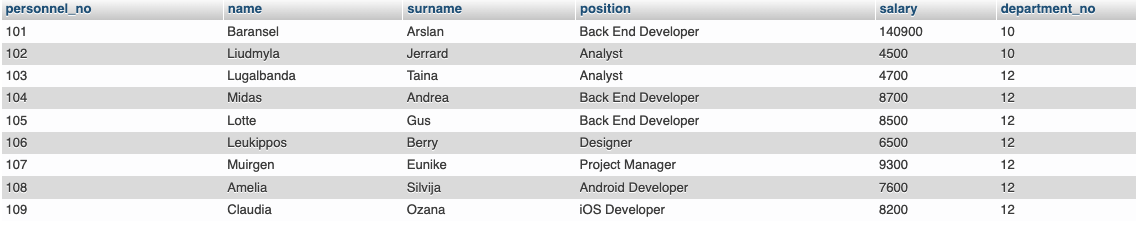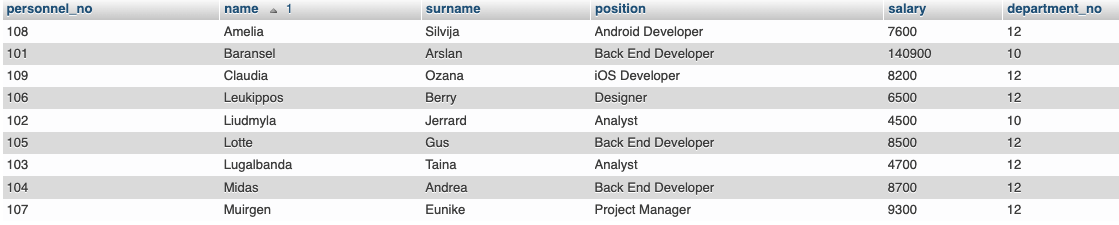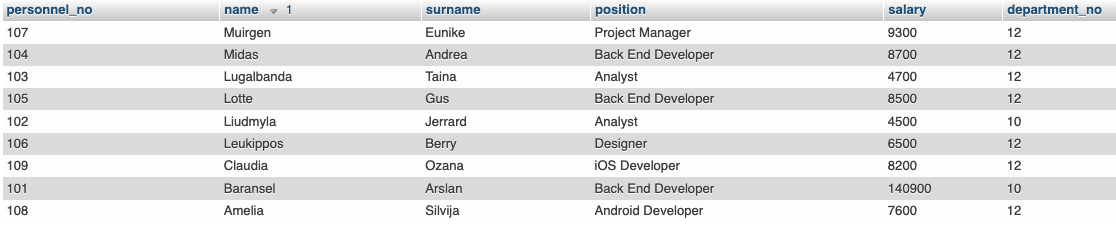The `ORDER BY` command, which is a SQL command, is the command used to obtain ordered tables. Let’s take a look at the table we created in our previous posts.Since the table here is small, we don’t have much trouble when we search for a data in the table, but when the number of people here increases, it will be very difficult to find these people in the list. For this, let’s sort the list in a certain order. For example, let’s sort them in alphabetical order. The keyword we will use when making SQL queries is the `ORDER BY` keyword. Let’s show it with an example.

``````SELECT * FROM company ORDER BY name
``````

While doing this, we write the name of the column according to which we will sort. Here, I wrote the `name` column because I want to sort by name.As you can see, the table is sorted in alphabetical order A-Z, if the column we want to sort was a numerical column instead of an alphabetical one, this time it would sort 0-9. Let’s sort it like this.

``````SELECT * FROM company ORDER BY name ASC
``````

As you can see, we got the same sorting again. So what is this `ASC` keyword? This keyword indicates that we will sort the list in ascending order. Now you will say that we didn’t use the `ASC` keyword in the first use, but again sorted in ascending order. True because SQL defaults to ascending order if you don’t specify it. So what do we do for reverse sorting? We will use the `DESC` keyword in it. Let’s show it now.

``````SELECT * FROM company ORDER BY name DESC
``````As you can see, this time it sorted Z-A. If it was a numeric column, it would sort 9-0.